# Magnetic Dipole Moment

## Magnetic Dipole Theory and Magnetic Potentials

To understand magnetic dipole moment, you first need to understand the simple magnetic moment.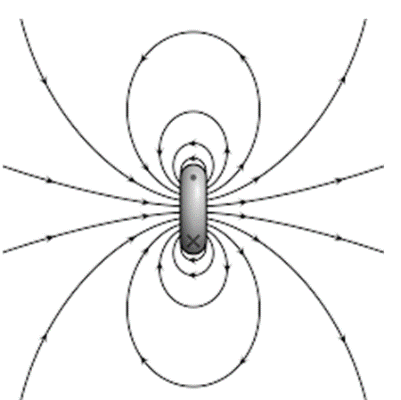Magnetic movement:

It is the magnitude that signifies the magnetic orientation and strength of a magnet or other object that creates a magnetic field. Examples of such objects that have magnetic moments contain loops of electric current (like electromagnets), elementary particles (such as electrons), permanent magnets, various molecules, and several astronomical objects (such as various planets, certain moons, stars, etc).

Exactly, the word magnetic moment normally denotes to a system's magnetic dipole moment, the component of the magnetic moment that can be denoted by the same magnetic dipole: magnetic north and south pole divided by a very minor distance. The magnetic dipole component is enough for small adequate magnets or for large sufficient distances. Higher order expressions (such as the magnetic quadrupole moment) can be needed in addition to the dipole moment for prolonged objects.

The magnetic dipole moment of an object or thing is readily defined in relations of the torque that object experiences in a certain magnetic field. The same applied magnetic field generates larger torques on objects with bigger magnetic moments. The direction and strength of this torque depend not only on the degree of the magnetic moment but also on its location relative to the direction of the magnetic field. The magnetic moment can also be considered, so, to be a vector.

Definition

It can be defined as a vector linking the aligning torque on the object from an outside applied a magnetic field to the field vector itself. The relationship is written by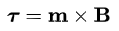Where τ is the torque acting on the dipole, B is the outside magnetic field, and m is the magnetic moment.

This definition is based on principle, of measuring the magnetic moment of an unknown sample. For a current loop, this definition hints to the magnitude of the magnetic dipole moment corresponding to the product of the current times the region of the loop. More, this definition agrees to the calculation of the projected magnetic moment for any known macroscopic current sharing.

A substitute definition is helpful for thermodynamic calculations of the magnetic moment. In this definition, the magnetic dipole moment of a structure is the negative gradient of its fundamental energy, Uint, with respect to the outer magnetic field: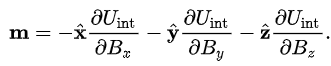Magnetic dipole.

It is the magnitude that signifies the magnetic orientation and strength of a magnet or other object that yields a magnetic field. Exactly, a magnetic moment mentions to a magnetic dipole moment, the constituent of the magnetic moment that can be signified by a magnetic dipole. A magnetic dipole is a magnetic north pole and South Pole divided by a minor distance.
Magnetic dipole moments have sizes of current time’s region or energy separated by magnetic flux density. The unit for dipole moment in centimeter–gram–second electromagnetic system, in meter–kilogram– second–ampere is an ampere-square meter, is the erg (unit of energy) per gauss (unit of magnetic flux density). One thousand ergs per gauss equal to one ampere-square meter.

The theory underlying in the magnetic dipole

The field (magnetic) of any magnet can be exhibited by a series of terms for which every term is more complicated (having finer angular feature) than the one before it. The first three terms of that series are known as monopole (denoted by isolated magnetic south or north pole) the dipole (denoted by two equal and opposite magnetic poles), and the quadrupole (denoted by four poles that combine together form two equal and opposite dipoles). The degree of the magnetic field for every term reduces progressively sooner with distance than the previous term so that at big enough distances the first non-zero term will govern.

For several magnets, the first non-zero word is the magnetic dipole moment. (To date, no isolated magnetic monopoles have been experimentally identified.) A magnetic dipole is the boundary of either a current loop or a pair of poles as the dimensions of the source are drop to zero while keeping the moment continuous. As long as these restrictions only apply to fields far from the sources, they are the same. However, the two models give different predictions for the inside field

Magnetic potentials

Usually, the equations for the magnetic dipole moment (and higher order terms) are derived from theoretical quantities known as magnetic potentials which are simpler to deal with mathematically than the magnetic fields.
In the magnetic pole model, the related magnetic field is the demagnetizing field H{\displaystyle \mathbf {H} }. Then the demagnetizing portion of H does not include, by description, the part of H {\displaystyle \mathbf {H} }due to free currents, there occurs a magnetic scalar potential such that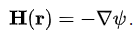In the amperian loop model, the related magnetic field is the magnetic induction B{\displaystyle \mathbf {B} }. Since magnetic monopoles do not occur, there happens a magnetic vector potential such that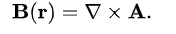Both of these potentials can be measured for any arbitrary current sharing (for the amperian loop model) or magnetic charge distribution (for the magnetic charge model) is providing that these are restricted to a small adequate region to give: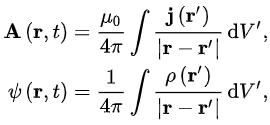Here p is the magnetic pole strength density in analogy to the electric charge density J is the current density in the amperian loop model, that leads to the electric potential, and the volume (triple) integrals over the coordinates that make up r’. The denominators of this equation can be prolonged with the help of multipole expansion to give a sequence of terms that have greater power of distances in the denominator. The first nonzero term, so, will dominate for great distances. The first non-zero term for the vector potential is given by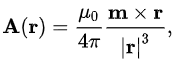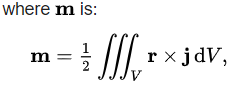Here r is the position vector, j is the electric current density & the integral is a volume integral.
× is the vector cross product, In the magnetic pole perspective, and the first non-zero term of the scalar potential is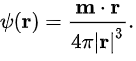Here m may be represented in terms of the magnetic pole strength density but is more usefully expressed in terms of the magnetization field as: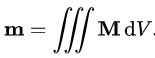The same symbol {\displaystyle \mathbf {m} }m is used for both equations since they produce equivalent results outside of the magnet.

Derivation of Magnetic Dipole Moment Formula

Magnetic Dipole moment-

The magnetic field,  R at a distance l along its axis, B due to a current loop carrying current i of radius, is given by: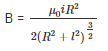At the present, if we think a point which is far from the current loop such that l>>R, then we can estimate the field as: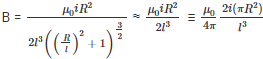currently, the area of the loop, A is
A = πR2

therefore, the magnetic field can be written as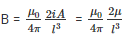We can mark this new quantity μ as a vector which points next to the magnetic field, so that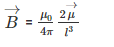take in the astounding connection to the  electric dipole field: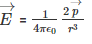Thus we call this quantity μ→ the magnetic dipole moment. Different electric fields such as magnetic fields do not have ‘charge ‘counterparts. Hence there are no sources or sinks of magnetic fields, here can only be a dipole. Whatever we produce a magnetic field comes with both a sink and a source that is there is both a north pole and south pole. With the help of different ways, the magnetic dipole is the fundamental unit that can produce a magnetic field.

Most elementary particles behave fundamentally as magnetic dipoles. For instance, the electron itself behaves as a magnetic dipole and has a Spin Magnetic Dipole moment. This magnetic moment is inherent from the electron has neither an area A (it is a point object) nor does it spin around itself but is fundamental to the nature of the electron’s existence.
We can simplify the magnetic moment for ‘N’ turns of the wire loop as
μ = NiA
The magnetic field lines of a current loop look alike to that of an idealized electric dipole:

The internal magnetic field of a dipole

The two types of the dipole (current loop and magnetic poles) give the same prediction for the magnetic field far from the source. On the other hand, inside the source region, they give different predictions. The magnetic field between both poles (see figure for Magnetic pole definition) is in the opposite direction to the magnetic moment (which points from the negative charge to the positive charge), while within a current loop it is in the same direction (see the figure to the right). The restrictions of these fields must also be diverse as the sources minimize to zero sizes. This difference only matters if the dipole limit is used to analyze fields inside a magnetic material.

If a magnetic dipole is shaped by making a present loop smaller and smaller, but keeping the product of current and area even, the limiting field is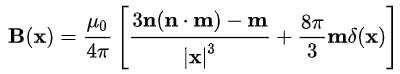Contrasting the expressions in the previous section, this limit is exact for the internal field of the dipole.
If a magnetic dipole is formed by taking a "north pole" and a "south pole", bring them closer and closer together but keeping the product of magnetic pole charge and distance constant, the limiting field is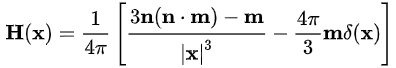These fields are connected by B = μ0(H + M), where M(x) = mδ(x) is the magnetization